This document is a part of the MATLAB Live Scripts set ‘Fatigue calculation in MATLAB' prepared by Adam Niesłony https://a.nieslony.po.edu.pl

# SN curve

## Introduction

### About the SN curve

The SN curve is used to provide basic information about the fatigue behavior of a material, simple element or machine part. In the name, S stands for stress and N stands for the cycle number to failure. Typically such a curve is presented on a log-log scale and represents 50% of life, ie. 50% of the specimens will fail under the load of given stress amplitude and number o cycle N. For the practical use of the curve in fatigue calculations, the actual shape of the graph has been simplified by using at least two straight lines in the log-log system. The basic plot, called also the Wohler curve, consists of two lines: one inclined for finite fatigue life and the other horizontal for infinite fatigue life.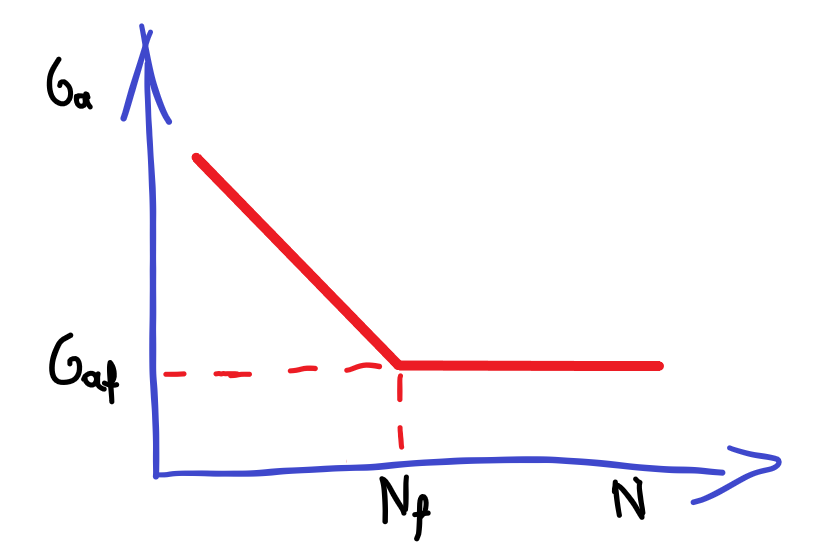Fig. 1. Simple SN curve with fatigue limit

### Mathematical forms used for SN curve

The general mathematical form of the SN curve is
Eq. (1)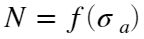where stress amplitude is the independent variable and the number of cycles is the dependent variable. The finite-life part of the SN curve, or the sloping section, is simply described by the following formula
Eq. (2)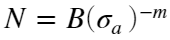where B and m are materail constants. The part of the curve parallel to the horizontal axis relates to the unlimited life and is usually defined by the fatigue limit (fatigue strength)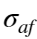value and the number of cycles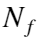for which this fatigue limit first appears. These are also material constants that can be used directly to describe the SN curve
Eq. (3)The simple form of the formula allows it to be transformed to obtain the stress amplitude as a function of the number of cycles
Eqs. (4-5)orIt is very practical to represent the SN curve with two lines, but this is a far-reaching generalization. In order to present an SN curve close to the real one, the segment method, in which the curve points are given directly and connected by straight lines in a double logarithmic system, is increasingly used. For example in FEM and CAD programs like ANSYS or SolidWorks this is the main method. To create such a plot, at least two pairs of stress amplitude - number of cycles should be specified, i.e.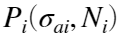for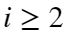. The first point can be the static strength for 0.5 load cycle. This method does not use the fatigue limit concept, so there should be no lines parallel to the horizontal axis. Instead, you can define the last line with a slight slope to the 'cycle axis'. During fatigue calculations, this segment is also used to extrapolate the SN curve.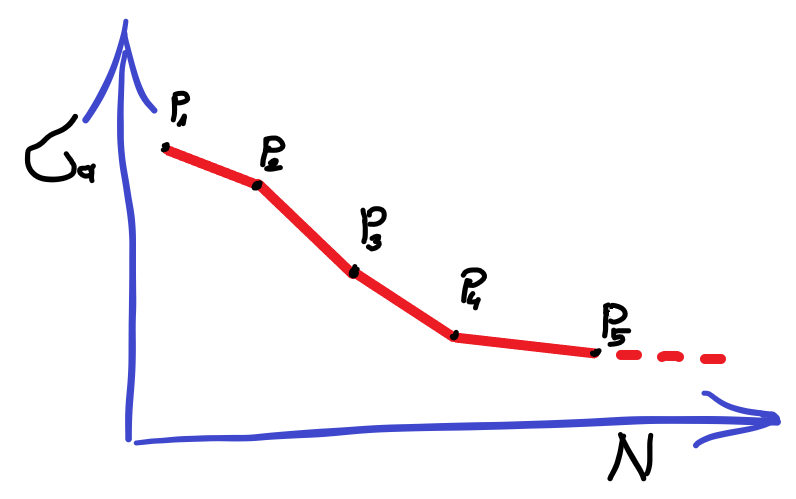Fig. 2. SN curve defined by points - the segment method

## Ploting of SN curve

### SN curve with fatigue limit

To plot the SN curve, we need basic material constants. It is best to use constants that have a physical meaning like fatigue limitand number of cycleat which the fatigue limit begins, the so-called knee point. Of course, the slope of the curve m must also be specified.
Saf = 250; % MPa, fatigue limit
Nf = 2e6; % number of cycle for fatigue limit
m = 8; % slope of the SN curve
B = Nf/(Saf^-m); % SN curve constant
% points for the plot
Ncurve = [1e4 Nf 4e7];
Scurve = (Ncurve./B).^(1/-m);
Scurve(end) = Saf;
% limits for the axis
lim = [1e3 1e8 200 600];
% ploting the curve in log-log scale with some annotation
h=loglog(Ncurve, Scurve,'k',...
[lim(1) Nf Nf],[Saf Saf lim(3)],'k--');
set(h(1),'LineWidth',2)
axis(lim)
xlabel('N, cycle')
ylabel('\sigma_{a}, MPa')
legend('SN curve')
x = [0.6,0.5];
y = [0.6,0.5];
annotation('textarrow',x,y,'String','N=B(\sigma_a)^{-m}');
annotation('textbox',[0.2 0.25 0.1 0.1],'String','\sigma_{af}','EdgeColor','none');
annotation('textbox',[0.65 0.1 0.1 0.1],'String','N_{f}','EdgeColor','none');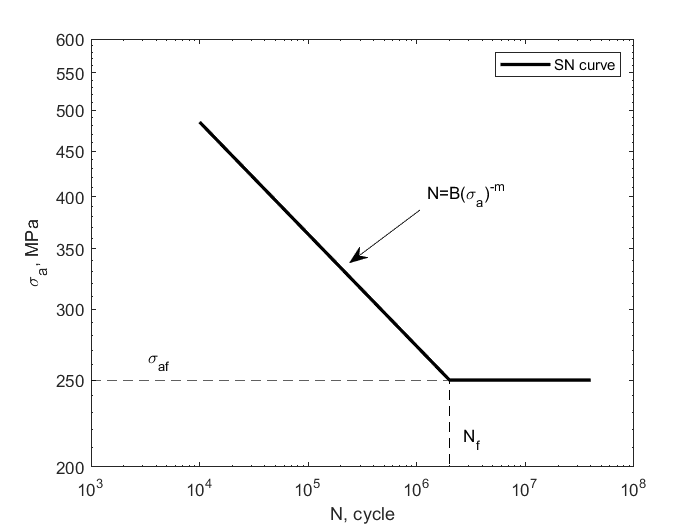### SN curve according the segment method

Drawing the SN curve according to the segment method is simple and consists in presenting individual pairs of points on the graph.
% points for segment method
Ncurve = [1e2 1e3 1e4 1e6 1e7 1e8];
Scurve = [550 520 450 290 260 250];
% limits for the axis
lim = [1e1 1e9 200 800];
% ploting the curve in log-log scale
h=loglog(Ncurve, Scurve,'ko-');
set(h,'LineWidth',2)
axis(lim)
xlabel('N, cycle')
ylabel('\sigma_{a}, MPa')
legend('SN curve')
% labeling the points
text(Ncurve,1.05*Scurve,{'P_1','P_2','P_3','P_4','P_5','P_6'})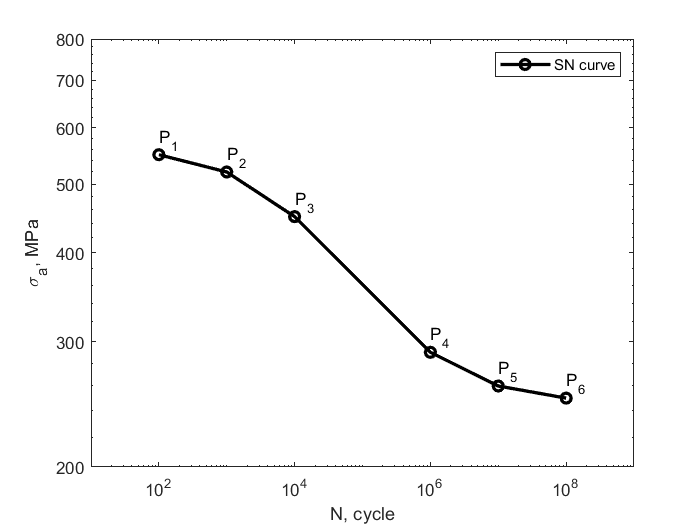## Reading values from the graph

### SN curve with fatigue limit

Having mathematical formulas describing the sloped part of the SN graph, it is easy to determine the number of cycles for given stress amplitude and vice versa. It should only be remembered that for some lower values of stress amplitude the cycle number reach infinity - it is the the so-called unlimited life range.
% Calculation the number of cycles
Sai = [500; 400; 300; 200];
Ni = zeros(size(Sai));
for i=1:length(Sai)
if Sai(i) > Saf
Ni(i) = B*Sai(i)^-m;
else
Ni(i) = inf;
end
end
disp(table(Ni,Sai))
Ni Sai __________ ___ 7812.5 500 46566 400 4.6514e+05 300 Inf 200
% Calculating the stres amplitude
Ni = [1000; 50000; 1e6; 5e7];
Sai = zeros(size(Ni));
for i=1:length(Ni)
if Ni(i)<Nf
Sai(i) = (Ni(i)/B).^(1/-m);
else
Sai(i) = Saf; % the maximum value for unlimited life
end
end
disp(table(Ni,Sai))
Ni Sai _____ ______ 1000 646.5 50000 396.46 1e+06 272.63 5e+07 250

### SN curve according the segment method

When reading the values from the segment plot, it should be remembered that all two adjacent points from SN curve should be connected with the function represented by the Eq. (2). Therefore, the constants m and B should be determined for each segment. However, there is a simpler method that uses interpolation. You only need to linearize the SN curve by taking the logarithm of the values that define the points of the graph. Then linear interpolation will give us intermediate points. In addition, you can apply extrapolation and points outside the defined SN curve will be extrapolated using the first and last segment.
% Calculation the number of cycles by given stres aplitudes
% point for the SN curve
Ncurve = [1e2 1e3 1e4 1e6 1e7 1e8];
Scurve = [550 520 450 290 260 250];
% stres amplitude
Sai = [560; 500; 400; 300; 200];
Nilog = interp1(log10(Scurve), log10(Ncurve), log10(Sai),'linear','extrap');
Ni = 10.^Nilog;
disp(table(Ni,Sai))
Ni Sai __________ ___ 47.726 560 1867.5 500 34368 400 7.0094e+05 300 4.8914e+13 200
% Calculation the stres aplitude by given number of cycles
% number of cycle
Ni = [10; 1000; 50000; 1e6; 2e8];
Sailog = interp1(log10(Ncurve), log10(Scurve), log10(Ni),'linear','extrap');
Sai = 10.^Sailog;
disp(table(Ni,Sai))
Ni Sai _____ ______ 10 581.73 1000 520 50000 385.95 1e+06 290 2e+08 247.07
End of file